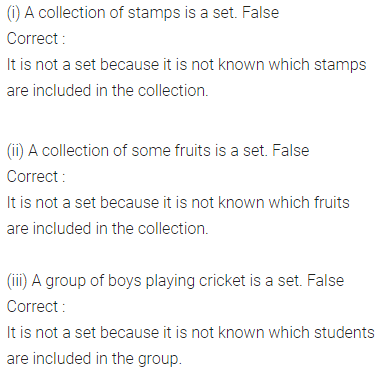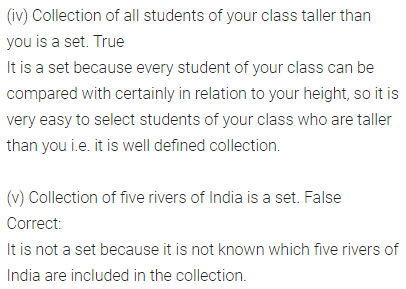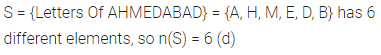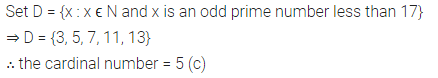# ML Aggarwal Class 6 Solutions for ICSE Maths Chapter 5 Sets Objective Type Questions

## ML Aggarwal Class 6 Solutions for ICSE Maths Chapter 5 Sets Objective Type Questions

Mental Maths
Question 1.
Fill in the blanks:
(i) A collection of ……… objects is called a set.
(ii) If x is a member of the set A, we write it as ………
(iii) The order of listing the elements of a set can be ………
(iv) If one or more elements are repeated, the set remains ………
(v) If X is the set of all letters in the word ‘MATHEMATICS’, then the cardinal number of the set X is ………
Solution:Question 2.
State whether the following statements are true (T) or false (F). Justify your answer.
(i) A collection of stamps is a set.
(ii) A collection of some fruits is a set.
(iii) A group of boys playing cricket is a set.
(iv) Collection of all students of your class taller than you is a set.
(v) Collection of five rivers of India is a set.
Solution:Multiple Choice Questions
Choose the correct answer from the given four options (3 to 9):
Question 3.
Which of the following collections is a set?
(a) Collection of all tasty fruits
(b) Collection of all good football players of your school
(c) Collection of all months of a year
(d) Collection of 5 most intelligent students of your class.
Solution:Question 4.
The tabular form of the statement ‘All months of a year whose names begin with the letters J’ is
(a) {January, June, July}
(b) {months of a year whose names begin with the letter J}
(c) {x | x is a month of a year whose name begins with the letter J}
(d) none of these
Solution:Question 5.
The method of representation used in the set A = {x | x is an even natural number less than 15} is called
(a) Description method
(b) Rule method
(c) Roster method
(d) None of these
Solution:Question 6.
The cardinal number of the empty set is
(a) 2
(b) 1
(c) 0
(d) none of these
Solution:Question 7.
If S = {x | x is a letter in the word AHMEDABAD}, then the cardinal number of S is
(a) 9
(b) 8
(c) 7
(d) 6
Solution:Question 8.
If A = {x : x ϵ N and x is an odd prime number less than 17}, then the cardinal number of A is
(a) 8
(b) 6
(c) 5
(d) none of these
Solution:Question 9.
{months of a year whose names begin with the letter F} is
(a) an infinite set
(b) empty set
(c) singleton set
(d) none of these
Solution: